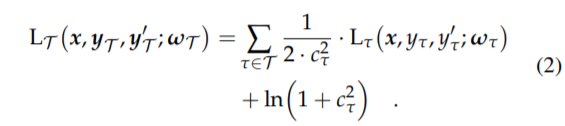# Loss function with learnable parameters

Let’s say my loss function contains learnable parameters, for example the weights to balance a multi objective loss function, how could I make sure the parameters in the loss function are trained?

Looking at the code in Learner, splitter is set to trainable_params by default which seems to take all trainable parameters from the model:

``````def trainable_params(m):
"Return all trainable parameters of `m`"
return [p for p in m.parameters() if p.requires_grad]
``````

Could I simply make a new splitter function that also include the parameters from the loss function?

Thanks!

You should include everything that’s trainable in your model in general. You can have the same tensor be a part of the model (that is unused) and the loss function (that is used there), since Tensors are a reference type, changing one/updating one will affect the other.

2 Likes

Makes sense! So the learned parameters tensors would simply be output of the model and passed to the loss function.

Thanks!

Did you manage to implement that loss function?

It’s been a long time since I worked on that. But I implemented this learnable weights method from the paper Auxiliary Tasks in Multi-task Learning. See section 2 for the formula.Here is how I implemented that in my code on a toy project. loss_weights are coming from model in fastai (returned by the model to the loss). I append the loss for each of the tasks I cared about in my problem in losses. Then applied the formula. From what I remember it learned sensible weights between the various task losses, but it`s been a long time since I looked at that code.

``````losses = []
losses += [self.l2(result_output, target)]
losses += [self.l2(question_output, question_target[:, 2])]
losses = torch.stack(losses)

losses = ((1/(2*(loss_weights**2))) * losses) + torch.log(1 + loss_weights**2)

return losses.sum()``````
1 Like

I’m trying to do something similar but I dont want to rewrite the AWD_LSTM to include the learnable parameters. I wonder if there’s a way to add new parameters to the AWD_LSTM model.

I don’t have the code in front of me, but you could simply subclass the AWD_LSTM model, in the constructor, create your learnable weights, in the forward function return the value of the base forward method and also your learnable weights. Eventually those two values would get passed down to your loss function where you can use them. From what I remember though AWD_LSTM uses a bunch of callbacks that might get affected if you do that.